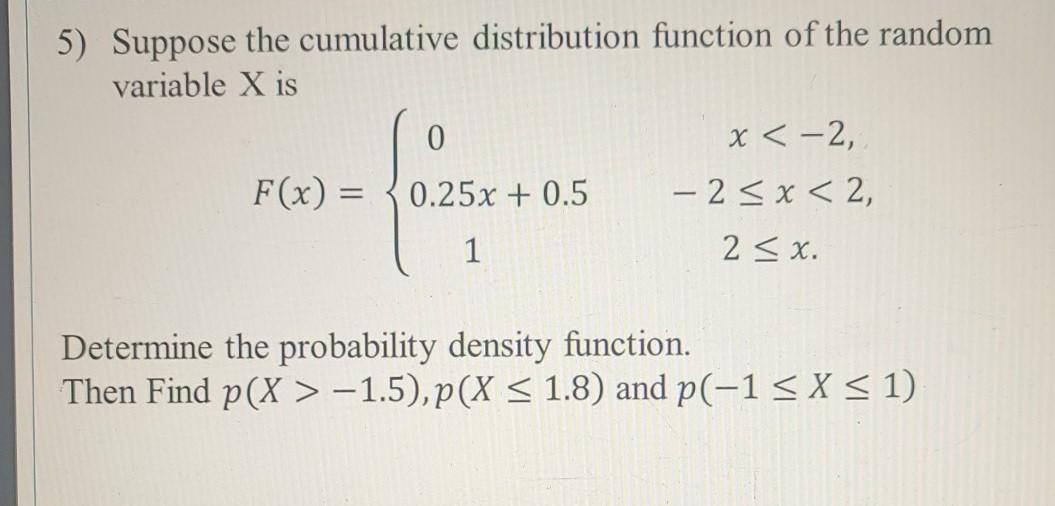# Question answer all.. 5) Suppose the cumulative distribution function of the random variable X is 0 x < -2, F(x) = 0.25x + 0.5 - 2 < x < 2, 1 2 < x. Determine the probability density function. Then Find p(X > -1.5),p(X < 1.8) and p(-1 < X < 1)